IMO Shortlist 2015 problem G5

Kvaliteta:
Avg: 2,0
Težina:
Avg: 8,0

Let$ABC$ be a triangle with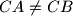$CA \neq CB$. Let$D$,$F$, and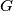$G$ be the midpoints of the sides$AB$,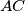$AC$, and$BC$ respectively. A circle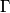$\Gamma$ passing through$C$ and tangent to$AB$ at$D$ meets the segments$AF$ and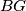$BG$ at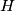$H$ and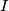$I$, respectively. The points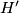$H'$ and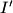$I'$ are symmetric to$H$ and$I$ about$F$ and$G$, respectively. The line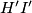$H'I'$ meets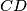$CD$ and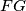$FG$ at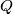$Q$ and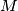$M$, respectively. The line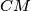$CM$ meets$\Gamma$ again at$P$. Prove that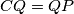$CQ = QP$.